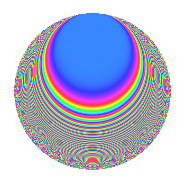# Properties

 Label 168.2.bcLevel 168 Weight 2 Character orbit bc Rep. character $$\chi_{168}(37,\cdot)$$ Character field $$\Q(\zeta_{6})$$ Dimension 32 Newform subspaces 1 Sturm bound 64 Trace bound 0

# Related objects

## Defining parameters

 Level: $$N$$ = $$168 = 2^{3} \cdot 3 \cdot 7$$ Weight: $$k$$ = $$2$$ Character orbit: $$[\chi]$$ = 168.bc (of order $$6$$ and degree $$2$$) Character conductor: $$\operatorname{cond}(\chi)$$ = $$56$$ Character field: $$\Q(\zeta_{6})$$ Newform subspaces: $$1$$ Sturm bound: $$64$$ Trace bound: $$0$$

## Dimensions

The following table gives the dimensions of various subspaces of $$M_{2}(168, [\chi])$$.

Total New Old
Modular forms 72 32 40
Cusp forms 56 32 24
Eisenstein series 16 0 16

## Trace form

 $$32q + 2q^{2} - 2q^{4} - 16q^{8} + 16q^{9} + O(q^{10})$$ $$32q + 2q^{2} - 2q^{4} - 16q^{8} + 16q^{9} + 6q^{10} + 22q^{14} - 10q^{16} - 2q^{18} - 40q^{20} - 12q^{22} - 8q^{23} - 6q^{24} + 16q^{25} + 6q^{26} - 26q^{28} - 8q^{30} - 24q^{31} - 8q^{32} - 24q^{34} - 4q^{36} - 26q^{38} - 6q^{40} - 4q^{42} + 20q^{44} + 16q^{46} - 24q^{47} - 16q^{48} + 8q^{49} + 52q^{50} + 44q^{52} - 64q^{55} + 40q^{56} - 16q^{57} + 34q^{58} - 22q^{60} + 100q^{62} - 20q^{64} + 12q^{66} + 16q^{68} + 38q^{70} - 80q^{71} - 8q^{72} + 8q^{73} + 10q^{74} - 32q^{76} + 12q^{78} + 8q^{79} - 56q^{80} - 16q^{81} - 16q^{84} - 22q^{86} + 24q^{87} + 50q^{88} + 12q^{90} + 64q^{92} - 48q^{94} + 24q^{95} + 10q^{96} - 48q^{97} - 64q^{98} + O(q^{100})$$

## Decomposition of $$S_{2}^{\mathrm{new}}(168, [\chi])$$ into newform subspaces

Label Dim. $$A$$ Field CM Traces $q$-expansion
$$a_2$$ $$a_3$$ $$a_5$$ $$a_7$$
168.2.bc.a $$32$$ $$1.341$$ None $$2$$ $$0$$ $$0$$ $$0$$

## Decomposition of $$S_{2}^{\mathrm{old}}(168, [\chi])$$ into lower level spaces

$$S_{2}^{\mathrm{old}}(168, [\chi]) \cong$$ $$S_{2}^{\mathrm{new}}(56, [\chi])$$$$^{\oplus 2}$$

## Hecke Characteristic Polynomials

There are no characteristic polynomials of Hecke operators in the database